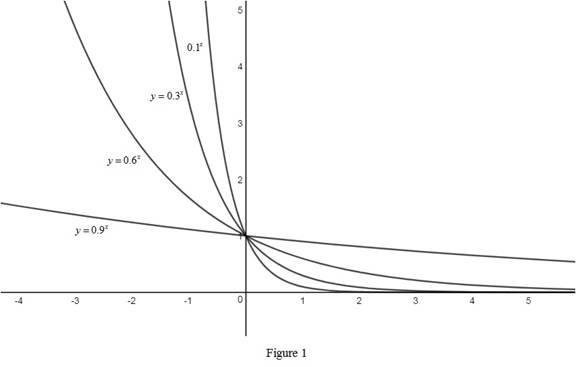# The graphs of the function y = 0.9 x , y = 0.6 x , y = 0.3 x and y = 0.1 x on the same axes and interpret how these graphs are related.### Single Variable Calculus: Concepts...

4th Edition
James Stewart
Publisher: Cengage Learning
ISBN: 9781337687805### Single Variable Calculus: Concepts...

4th Edition
James Stewart
Publisher: Cengage Learning
ISBN: 9781337687805

#### Solutions

Chapter 1.5, Problem 10E
To determine

## To sketch: The graphs of the function y=0.9x,y=0.6x,y=0.3x and y=0.1x on the same axes and interpret how these graphs are related.

Expert Solution

### Explanation of Solution

The rough graphs of the functions y=0.9x,y=0.6x,y=0.3x and y=0.1x are drawn and shown below in Figure 1.From Figure 1, it is observed that the graphs of the exponential functions whose base is greater than 0 but lesser than 1 are passing through the common point (0, 1) as any number raised to the power 0 is always 1.

Also, notice that the graphs approaches 0 as x tending to and approaches as x tending to . Therefore, the graphs are monotonically increasing.

### Have a homework question?

Subscribe to bartleby learn! Ask subject matter experts 30 homework questions each month. Plus, you’ll have access to millions of step-by-step textbook answers!